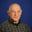Related Tags

java
parameters
class
equal

# How to define the equals method in a Java parameterized classFrank M. Carrano

### Overview

In another Answer, we defined the equals method for the class Name as follows:

public class Name
{
private String first; // First name
private String last;  // Last name
.
.
.
public boolean equals(Object other)
{
boolean result = false;
if (other instanceof Name)
{
Name otherName = (Name)other;
result = first.equals(otherName.first) &&
last.equals(otherName.last);
} // End if

return result;
} // End equals
} // End Name

The equals method in a class Name

Notice how we had to cast the equals method’s parameter other from type Object to Name. However, if our class is parameterized using a generic data type, we must take special care when making this cast.

### Equals method in a parametrized class

Consider the following example:

Driver.java
Pair.java
/** A driver that demonstrates the class Pair.
@author Frank M. Carrano */
public class Driver
{
public static void main(String[] args)
{
Pair<String> twoFruit = new Pair<>("apple", "orange");
System.out.println("A pair of fruit: " + twoFruit);

Pair<String> theSameTwoFruit = new Pair<>("orange", "apple");
System.out.println("The same pair of fruit: " + theSameTwoFruit);

Pair<String> twoOtherFruit = new Pair<>("banana", "grape");
System.out.println("Another pair of fruit: " + twoOtherFruit);

System.out.print("\nIs the pair " + twoFruit);
System.out.println(" equal to the pair " + twoOtherFruit + "?");
System.out.println(twoFruit.equals(twoOtherFruit) + " [Should be false]");

System.out.print("\nIs the pair " + twoFruit);
System.out.println(" equal to the pair " + twoFruit + "?");
System.out.println(twoFruit.equals(theSameTwoFruit) + " [Should be true]");

System.out.print("\nIs the pair " + twoFruit);
System.out.println(" equal to the pair " + theSameTwoFruit + "?");
System.out.println(twoFruit.equals(theSameTwoFruit) + " [Should be true]");
} // End main
} // End Driver
An equals method in a parameterized class

Let’s closely examine the highlighted lines in this copy of the equals method:

public boolean equals(Object other)
{
boolean result = false;
if (other instanceof Pair<?>)
{
Pair<?> otherPair = (Pair<?>)other;
result = (first.equals(otherPair.first) && second.equals(otherPair.second)) ||
(first.equals(otherPair.second) && second.equals(otherPair.first));
} // End if

return result;
} // End equals

If Pair were not parameterized, line 4 would be as follows:

if (other instanceof Pair)


And line 6 would be as follows:

Pair otherPair = (Pair)other;


But our class Pair is parameterized. The occurrence of Pair in lines 4 and 6 is flagged as a raw type. Note that we should not use raw types in a parameterized class.

Now, instead, let’s consider that line 4 is written as follows:

if (other instanceof Pair<T>)


In this case, we would get this syntax error message:

error: illegal generic type for instanceof


Now, let’s consider that line 6 is written as follows:

Pair<T> otherPair = (Pair<T>)other;


In this case, we would get this warning:

warning: [unchecked] unchecked cast


The solution is to write the wildcard ? instead of the generic data type T, as we have done in the equals method.

Note that the statement below

Pair<?> otherPair = (Pair<?>)other;


works because we access otherPair’s fields without changing their values, and we do not call otherPair’s methods. In other cases, using ? may not avoid the warning of an unchecked cast.

RELATED TAGS

java
parameters
class
equal

CONTRIBUTORFrank M. Carrano
RELATED COURSES

View all Courses

Keep Exploring

Learn in-demand tech skills in half the time